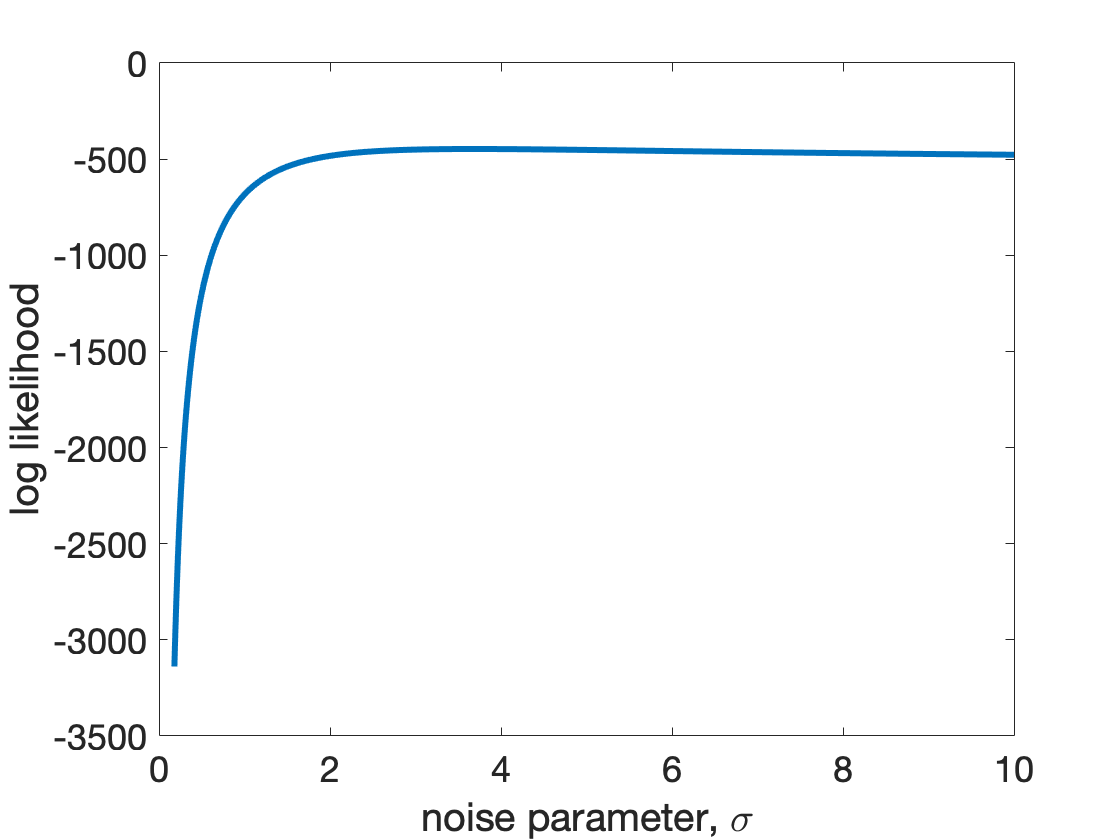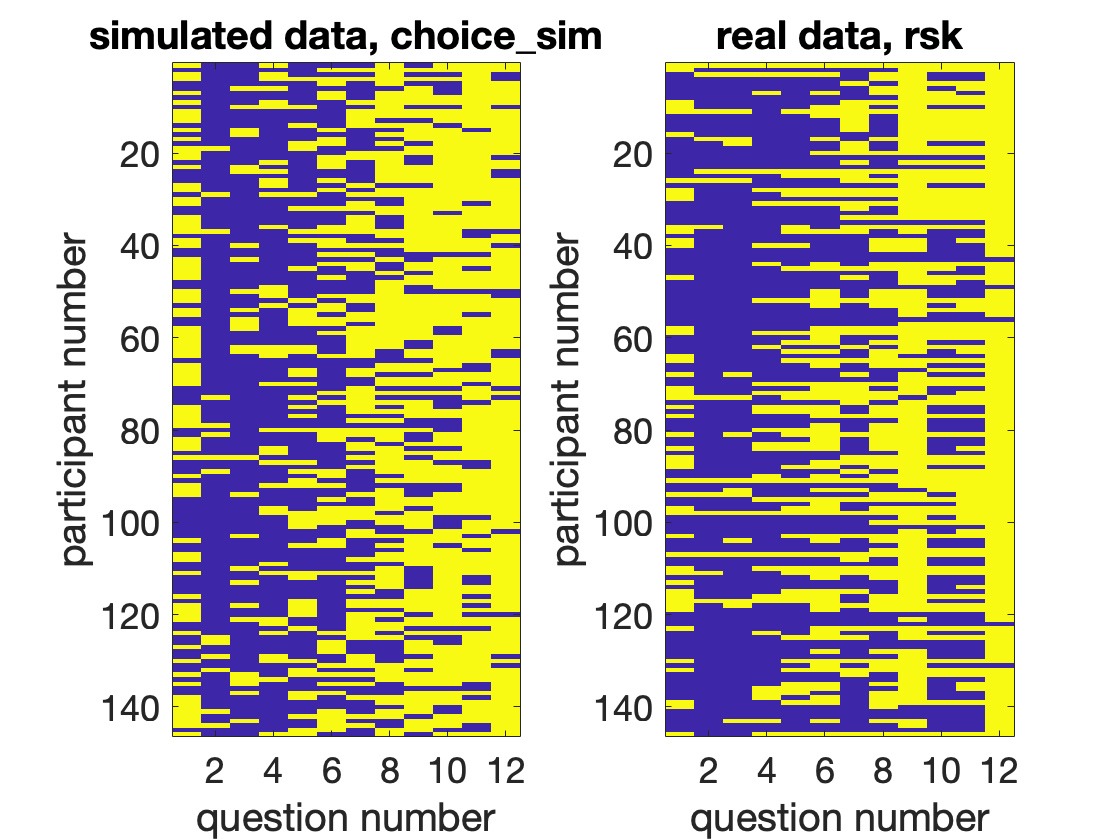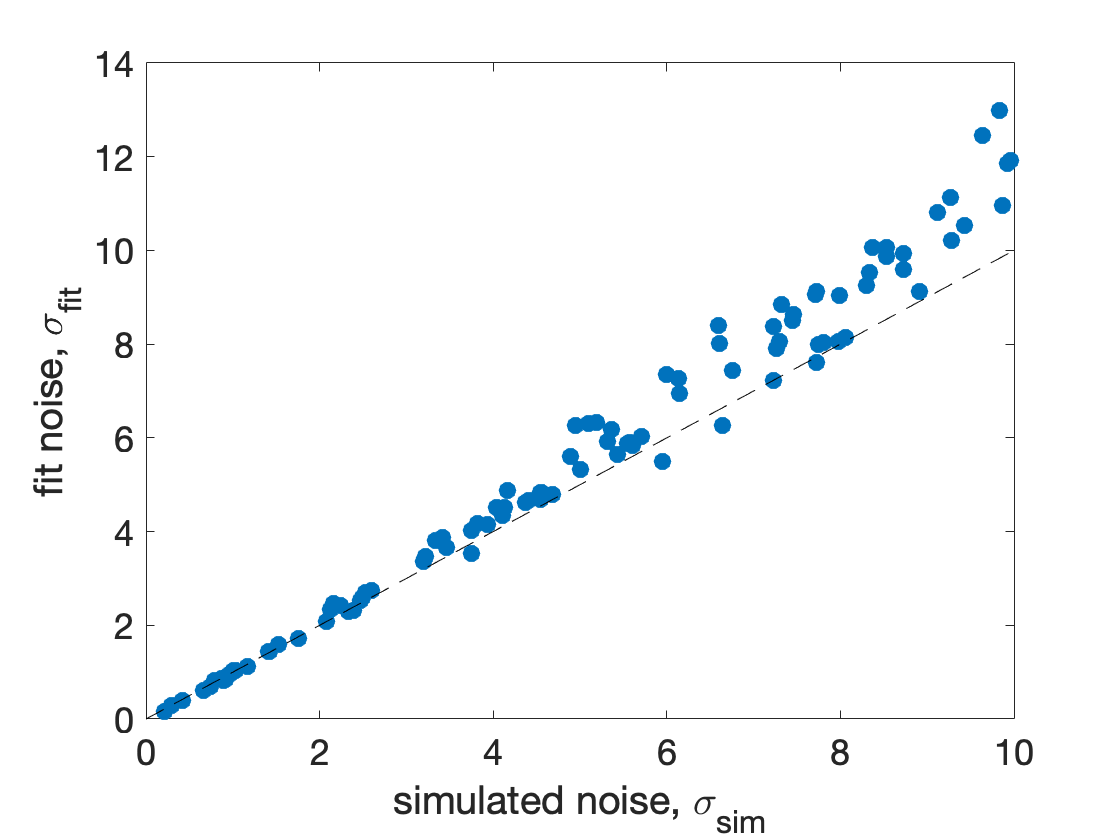# Maximum likelihood model fitting and parameter recovery

Last week we fit the softmax model using a Bayesian approach. This was more complicated than the least squares model fitting we did in Week 8, but has the advantage that we can estimate the uncertainty in our fit parameters.
However, fully Bayesian methods run into difficulty as the number of parameters in our model increases, because to compute the posterior distribution we need to evaluate the likelihood at all possible values of all the parameters - a calculation that gets exponentially more difficult the more parameters we have.
In part for this reason, we often use approximations to the Bayesian approach in practice. Indeed what we did last week was an approximation because we only evaluated the posterior on a "grid" of possible sigma values not all sigma values up to infinity! There are many, many different ways to approximate Bayesian parameter fitting and it's an active area of research to figure out which approximations are the best and in which conditions. These approximations can also be quite complicated and computationally intense (one approximation that we've worked with in our lab takes about 3 days to fit on a high end laptop).
However, there is one popular approach that is pretty easy and gives fairly good results in many cases. I have published several papers using this techique and while it's no longer "state of the art" it's good enough in many situations. This approximation is called the maximum-likelihood approach ...

## Maximum likelihood model fitting

The maximum likelihood approximation does exactly what it sounds like it does - it estimates the parameter value as the one that maximizes the likelihood. Because the log transformation preserves the location of the maximum, maximizing the likelihood is identical to maximizing the log likelihood, which is nice because, as we saw last week, the log likelihood is easier for Matlab to deal with.
So how do we maximize the likelihood? Well first, let's copy some code from last week and plot the likelihood as a function of sigma for our data set ...
clear
% compute expected values
EV_safe = 10;
EV_risky = P.*V;
% compute log likelihood as a function of sigma
sigma_vals = [0.01:0.01:10];
for i = 1:length(sigma_vals)
logLikelihood(i) = computeLogLikelihood(EV_safe, EV_risky, rsk, sigma_vals(i));
end
% plot the log likelihood
clf;
plot(sigma_vals, logLikelihood, 'LineWidth', 3)
xlabel('noise parameter, \sigma')
ylabel('log likelihood')
set(gca, 'fontsize', 18)You can already see from this plot that the maximum is around 3-4. How can we get Matlab to find this maximum automatically? At this point you may be hoping for a function fmaxcon - that automatically finds the maximum just like fmincon automatically finds the minimum, but Matlab won't give it to you. This is because maximizing a function is identical to minimizing the negative of that function. So we can use fmincon to minimize the negative log-likelihood.
One way to do this is to define our objective function handle like this ...
fHandle = @(x) -computeLogLikelihood(EV_safe, EV_risky, rsk, x);
Then I can just use fmincon like we did two weeks ago ...
% initial condition
X0 = 1;
% lower bound
LB = 0;
% upper bound
UB = inf;
% minimize the negative log likelihood
sigmaBest = fmincon(fHandle, X0, [], [], [], [], LB, UB)
Local minimum possible. Constraints satisfied. fmincon stopped because the size of the current step is less than the value of the step size tolerance and constraints are satisfied to within the value of the constraint tolerance. <stopping criteria details>
sigmaBest = 3.6744
Our maximum likelihood estimate for the parameter is 3.6744. And that's it for model fitting! But now that we know how to fit model parameters, there's one more thing we need to check to make sure that our the parameter fits we get actually have any meaning ...

## Parameter recovery

Fitting model parameters to data is great, and once you can do it, it's tempting to start to interpret the parameter values you get. For our simple model you could say things like - people with large sigma values have more noise in their decision process than people with low sigma values. Perhaps the estimated sigma values also correlate with biological factors like age or blink rate or the fMRI signal in some part of the brain. Or maybe people with schizophrenia or depression or anxiety have more noise than people without these conditions.
Such interpretation of numbers is exactly what scientists are trained to do, and doing it well moves science forwards. However, there's an extra step we need to do with parameter values we derive from model fits before we can be sure (or at least more sure) that our fit parameter values are valid.
In particular, it's possible with model fitting to get completely bogus parameter values. This can happen for all sorts of reasons ranging from a bug in your code to fundamental mathematical problems with your model to issues with your experiment. To test whether this is happening in your situation you can check for this problem by fitting simulated data, where we know what the real parameter values are. Then we can ask whether the fit parameter values match the simulated parameter values.
So what we are going to do is this:
• Simulate some data in our experiment using the noisy expected value theory model we developed in Week 6 with known values of the noise - we will call these simulated parameter values "sigma_sim"
• Fit the simulated data using the maximum likelihood approach we described above - call these fit parameter values "sigma_fit"
• Then we will ask whether sigma_sim = sigma_fit (or at least whether they are close).
• If the fit parameters are close to the simulated parameters, then we're in the clear. If they are not close then we need to go back to the drawing board.
Let's give it a go ...

## Simulating the data using code from Week 6

Copy over the two functions you made in Week 6 "NEVtheory" and "noisyExpectedValueTheory." Together, these functions allow you to simulate the model ...
% number of simulations per question
N = 146;
% simulated value of sigma
sigma_sim = 3;
% simulate!
for i = 1:length(P)
[~, ~, ~, choice(:,i)] = NEVtheory(N, EV_safe, P(i), V(i), sigma_sim);
end
Note: the ~ correspond to outputs of NEVtheory I just want to ignore.
This gives us a simulated data set (choice) that is similar to the real data set (rsk). The only difference is that choice is 1 when we choose the safe option, while rsk is 1 when we choose the risky option, to get them to line up we need one more step
choice_sim = 1 - choice;
Then plotting choice_sim and rsk side by side shows the broad similarities
clf;
subplot(1,2,1)
imagesc(choice_sim);
title('simulated data, choice_sim', 'interpreter', 'none')
xlabel('question number')
ylabel('participant number')
set(gca, 'fontsize', 18)
subplot(1,2,2)
imagesc(rsk);
title('real data, rsk')
xlabel('question number')
ylabel('participant number')
set(gca, 'fontsize', 18)## Fitting the simulated data using code from Week 10

Now we've got the simulated data, we can fit it using code from this week. We just have to replace rsk with choice_sim in the function handle ...
fHandle = @(x) -computeLogLikelihood(EV_safe, EV_risky, choice_sim, x);
and then the fitting code is the same
% initial condition
X0 = 1;
% lower bound
LB = 0;
% upper bound
UB = inf;
% minimize the negative log likelihood
sigma_fit = fmincon(fHandle, X0, [], [], [], [], LB, UB)
Local minimum possible. Constraints satisfied. fmincon stopped because the size of the current step is less than the value of the step size tolerance and constraints are satisfied to within the value of the constraint tolerance. <stopping criteria details>
sigma_fit = 3.1575
So the fit value is 3.1575, while the simulated value was 3. That's pretty good agreement! But is that just a fluke? To tell we will need to repeat this process of simulating and fitting multiple times and for different values of sigma ...

## Plotting sigma_fit vs sigma_sim

To make this process a bit easier, let's make a function for simulation
function choices = simulate(N, EV_safe, P, V, sigma)
% simulate!
for i = 1:length(P)
[~, ~, ~, choice(:,i)] = NEVtheory(N, EV_safe, P(i), V(i), sigma);
end
choices = 1 - choice;
and a function for fitting
function sigma_fit = fit(EV_safe, EV_risky, choice_sim)
% function handle
fHandle = @(x) -computeLogLikelihood(EV_safe, EV_risky, choice_sim, x);
% initial condition
X0 = 1;
% lower bound
LB = 0;
% upper bound
UB = inf;
% minimize the negative log likelihood
sigma_fit = fmincon(fHandle, X0, [], [], [], [], LB, UB)
Then let's run these in a loop with random values for sigma_sim each time ...
clear
EV_safe = 10;
N = 146; % simulated number of participants
for i = 1:100 % repeat 100 times
% set simulated sigma value randomly between 0 and 10
sigma_sim(i) = rand*10;
% simulate choices
choice_sim = simulate(N, EV_safe, P, V, sigma_sim(i));
% fit simulated choices
sigma_fit(i) = fit(EV_safe, P.*V, choice_sim);
end
Then finally we can plot the results like this ...
clf;
plot(sigma_sim, sigma_fit, '.', 'markersize', 30)
hold on;
plot([0 10], [0 10], 'k--') % put line of equality on there
xlabel('simulated noise, \sigma_{sim}')
ylabel('fit noise, \sigma_{fit}')
set(gca, 'fontsize', 18)This shows that there's a really close relationship between the simulated and fit parameters showing us that parameter is really good in this case. This means that the model works well when fitting data that are simulated by our model. Note that this is the absolute best case scenario for our model. For real data we don't actually know which model generated the data - because we don't know what computations people are actually performing. Thus, while good parameter recovery like this can give us some confidence in our model parameters, we should still be careful in how we interpret them because it could be that we have the wrong model to begin with!
Figuring out whether your model is good enough is an art form that goes beyond the scope of this class, but if you're interested, more information on (slightly) more advanced topics can be found in this paper.